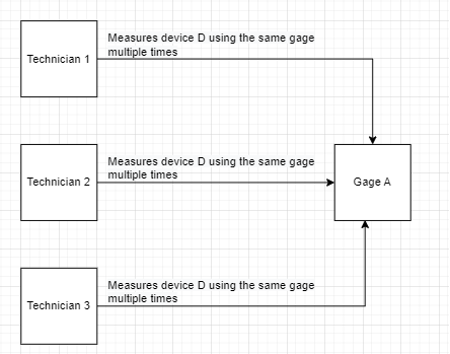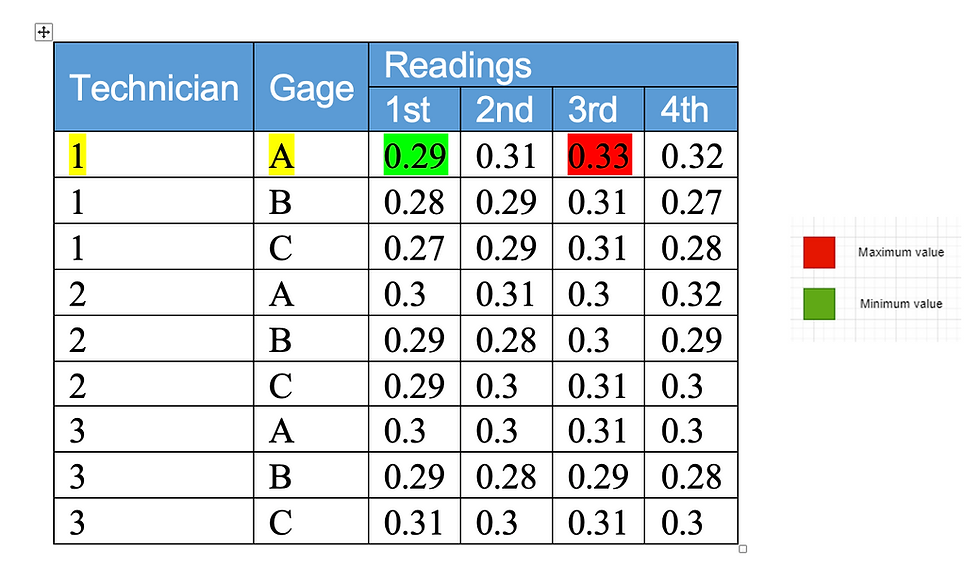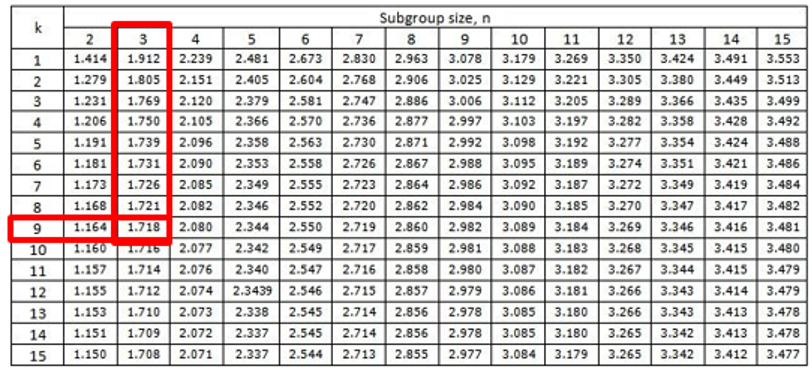top of page
Search
• Metquay Inc Consulting Team

# Introduction to Gage R&R Ver 2023

Updated: Feb 25

In the world of quality control, it is essential to gauge the validity of a measuring system and take necessary steps to ensure minimal process variation caused by a measuring system. Such a system, better known as Measurement System Analysis (MSA) is carried out across the industry to determine the acceptable amount of error in world-class standards.

MSA employs Precision as one of its key metrics in determining the quality of a measuring system. Measurements are deemed precise if they differ from one another by a very small amount. Precision can be defined as the degree to which repeated measurements, remain the same under the same conditions. Precision can also be explained as the closeness of two or more measurements to each other. Gage R and R are further tests carried out to further know how precise a measuring system is.

The first R stands for Repeatability, also known as equipment variation (EV), and let us understand the definition of this word with an example. Consider a scenario where a technician let's identify him as Technician 1, is measuring a device D using a gage, Gage A.

The same technician (Technician 1) is then made to measure the same part D, multiple times using the same Gage A under the same conditions and the readings are noted down. The variability amongst these repeated readings by the same technician gives us the Repeatability of the measuring system.Image 1.1 Repeatability

The next main concept is Reproducibility, also known as Appraiser Variation (AV).

Now, let us consider the same example, but this time let us consider 3 technicians (Technician A, Technician B, and Technician C) and they are made to measure the same device D, using Gage A. They are made to measure device D, multiple times under the same conditions. The variation in readings that occur, when different technicians are involved, is what is known as Reproducibility.Image 1.2 Reproducibility

Now, let us consider the same example, but this time let us consider 3 technicians (Technician A, Technician B, and Technician C) and they are made to measure the same device D, using Gage A. They are made to measure device D, multiple times under the same conditions. The variation in readings that occur, when different technicians are involved, is what is known as Reproducibility.Image 1.2 Reproducibility

Now let us take an example to see how to calculate the Repeatability and reproducibility of an instrument using the Average- Range Method.

Firstly, let us combine the readings of three Technicians named 1,2, and 3 who repeatedly measure Gages A, B, and C, and take 4 readings per each combination.For Technician 1 and Gage A, the Range = Max value – Min value = 0.33-0.29=0.04

For Technician 1 and Gage A, the Mean (Average of all readings combined) = (0.29+0.31+0.33+0.32)/4 = 0.3125

Similarly, let us compute the range and mean for all the possible combinations.The next step is to calculate the ‘mean of range’ and ‘Mean of mean’ for each Technician.

For Technician 1: the mean of range = (0.04+0.04+0.04)/3= 0.04

Similarly, the mean of mean of technician A = (0.3125+0.2875+0.2875)/3 = 0.2958

Similarly, compute the mean of range and mean of the mean of each of the technicians and note them.Now, to calculate Repeatability: Repeatability = R̅*k1

Where R̅ = Total mean range = 0.023 and k1 =1/d2

To find the k1 we need the d2 value, which can be found in the table (D2 values for the distribution of the average range) based on subgroup size and the number of combinations of parts and technicians.

In this example, the subgroup size = number of readings = 4

Number of the combination of parts (number of gages used) and technicians= 3 parts and 3 technicians = 3*3=9Therefore, our calculation readings after substituting values in the repeatability formula, come up to:

d2 = 2.08

k1 =1/d2 =0.480769231

Repeatability= EV = R̅*k1= 0.01122

To calculate Reproducibility (Appraiser Variation (AV)), we use the below formula:Where x̅diff is the range of means = 0.0033

Where n= number of parts (number of gages) = 3

To find the k2 we need the d2 value from the table (D2 values for the distribution of the average range); the d2 value can be found from the table based on the number of parts and number of combinations of parts and technicians (g)

Number of parts =3

Number of the combination of parts and technicians (g) = 3 parts and 3 technicians = 3*3=9Therefore, from the table the value of d2 =1.718

k2=1/d2 =1/1.718 = 0.5820If the number is calculated as a negative value, set Repeatability (AV)=0, so in this case, wherein AV=0.

Now to calculate the gage R and R, the formula used is:Where EV = Repeatability = Equipment variation

And AV = Reproducibility = Appraiser Variation

RR= 0.011217949 = 1.12%

According to the Automotive Industry Action Group (AIAG) For your measurement system assessment using %GRR:For the above example, the RR value lies in the green zone, hence making it an acceptable measurement system.

In equipment calibration, the most common process deviances can come up because of faulty equipment or irregularities in the process carried out by technicians. These two root causes are captured using Repeatability and Reproducibility.

This calculation mechanism of R and R provides an avenue to understand where the root cause is, and apply a fix to stay on top of your equipment and process quality and help take informed decisions, backed by mathematics!